# RD Sharma Solutions For Class 12 Maths Exercise 4.9 Chapter 4 Inverse Trigonometric Functions

Students can refer to RD Sharma Solutions for Class 12 Maths Exercise 4.9 Chapter 4 Inverse Trigonometric Functions which are available here. The solutions here are solved step by step for a better understanding of the concepts, which helps students prepare for their exams with ease. Our expert tutors at BYJU’S have made it possible to help students crack difficult problems. This exercise includes the properties of inverse trigonometric functions. To know more about this topic students can download RD Sharma Solutions for Class 12.

## Download the PDF of RD Sharma Solutions For Class 12 Chapter 4 – Inverse Trigonometric Functions Exercise 4.9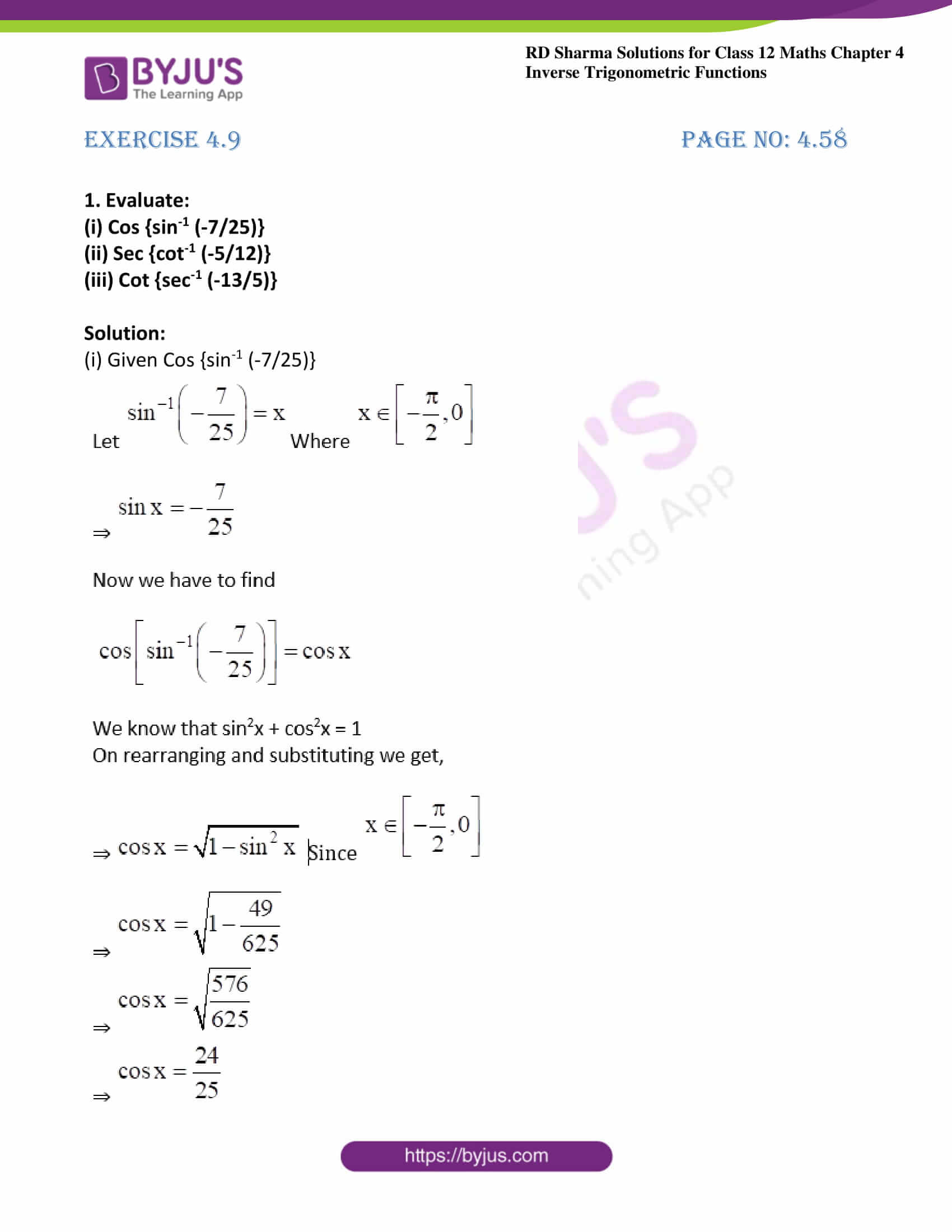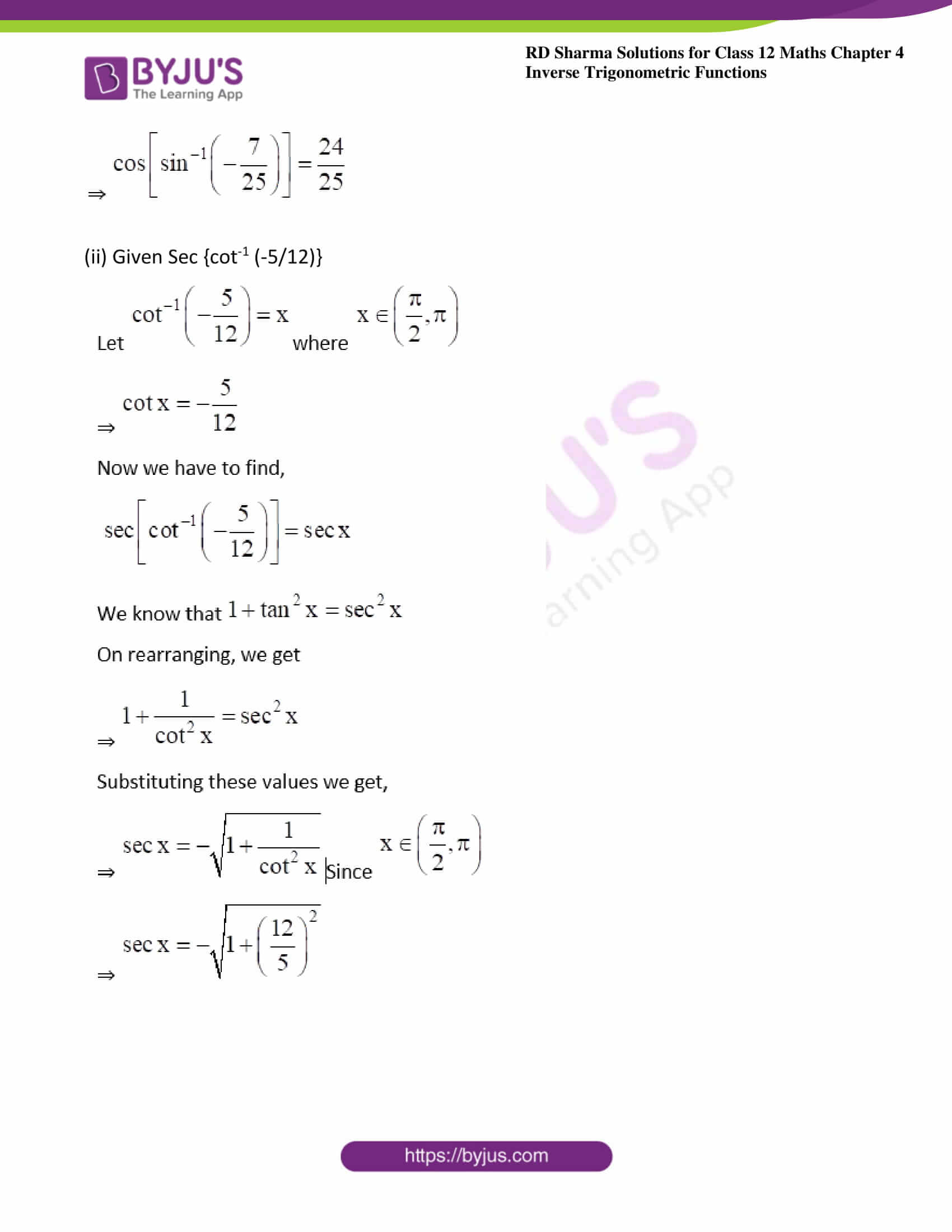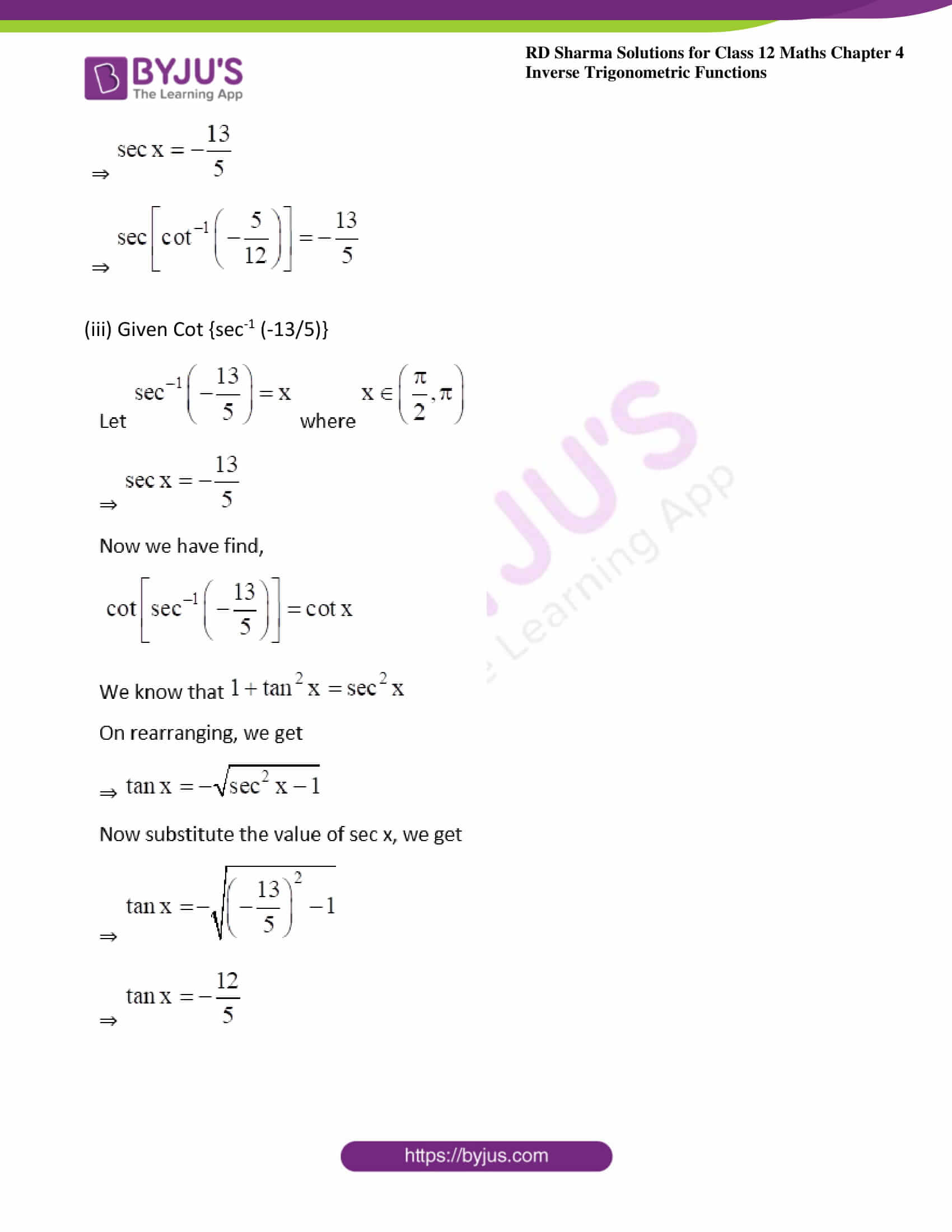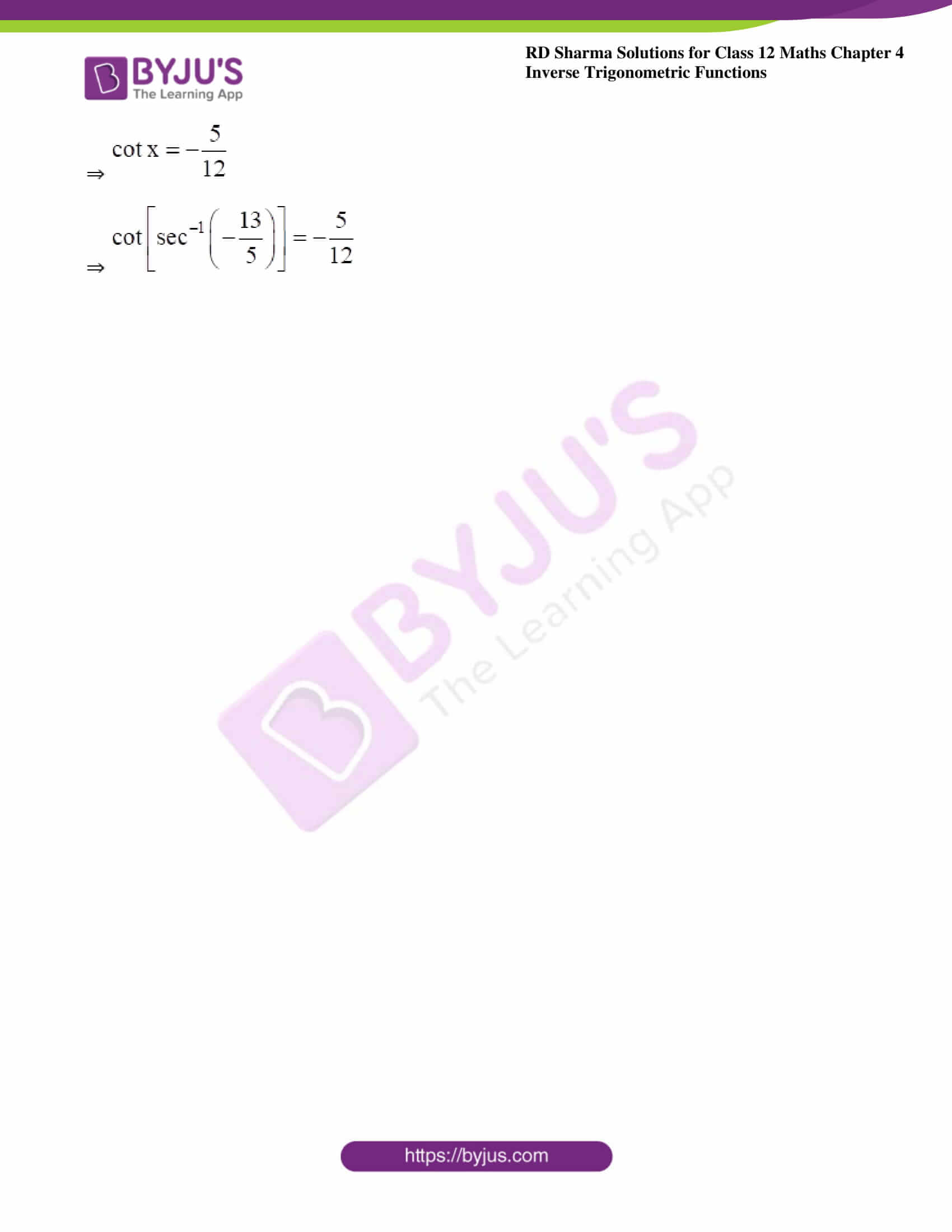### Exercise 4.9 Page No: 4.58

1. Evaluate:

(i) Cos {sin-1 (-7/25)}

(ii) Sec {cot-1 (-5/12)}

(iii) Cot {sec-1 (-13/5)}

Solution:

(i) Given Cos {sin-1 (-7/25)}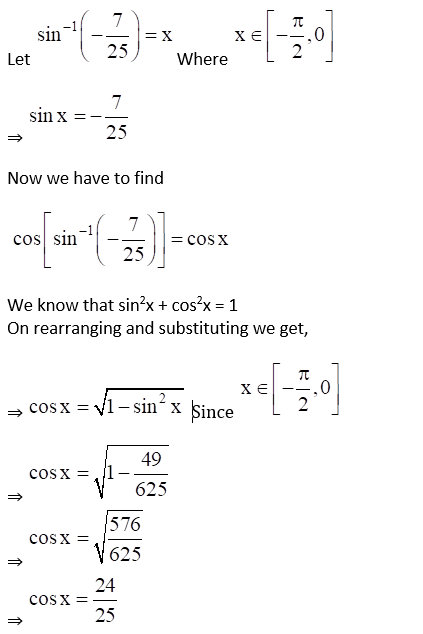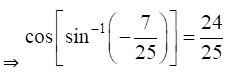(ii) Given Sec {cot-1 (-5/12)}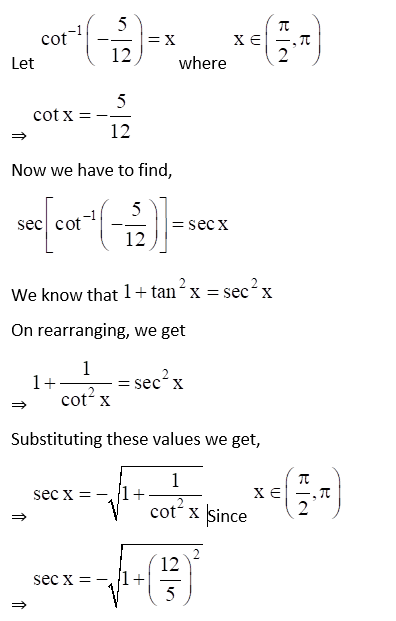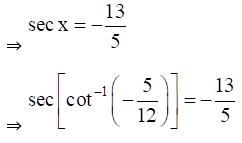(iii) Given Cot {sec-1 (-13/5)}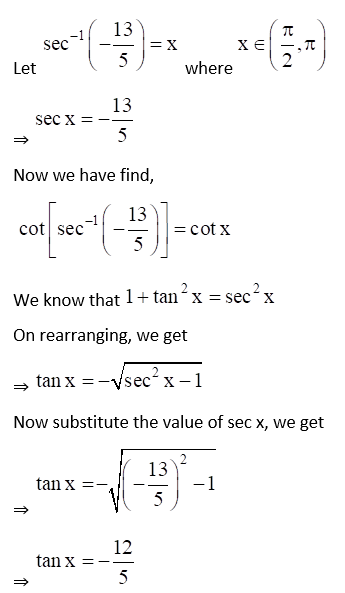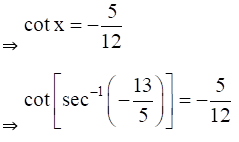### Access other exercises of RD Sharma Solutions For Class 12 Chapter 4 – Inverse Trigonometric Functions

Exercise 4.1 Solutions

Exercise 4.2 Solutions

Exercise 4.3 Solutions

Exercise 4.4 Solutions

Exercise 4.5 Solutions

Exercise 4.6 Solutions

Exercise 4.7 Solutions

Exercise 4.8 Solutions

Exercise 4.10 Solutions

Exercise 4.11 Solutions

Exercise 4.12 Solutions

Exercise 4.13 Solutions

Exercise 4.14 Solutions